# Simple interest And Compound interest for IBPS , SBI , RBI | Quantitative Aptitude | Quiz-5

## Simple interest And Compound interest for IBPS , SBI , RBI

Simple interest And Compound interest plays a significant role in Quantitative Aptitude Section of banking exams such as IBPS, SBI and RBI PO and Clerk. You will get at least 4-5 questions from Simple interest And Compound interest in one of IBPS, SBI and RBI PO & clerk exam. So, aspirants should focus on Simple interest And Compound interest questions in detail. Here, we are providing you with the Simple interest And Compound interest questions quiz with the detailed solution so that you can easily prepare for Simple interest And Compound interest questions. We are providing here all-important latest pattern-based questions and Previous Year Questions of Simple interest And Compound interest of various Government Exam like IBPS, SBI, and RBI PO and Clerk exam. This Simple interest And Compound interest quiz we are providing is free. Attempt this Simple interest And Compound interest quiz to practice important questions with answers and solutions. And score better in IBPS, SBI and RBI PO and Clerk exam.

Simple interest And Compound interest Quiz to improve your Quantitative Aptitude for SBI Po & SBI clerk exam IBPS PO Reasoning , IBPS Clerk Reasoning , IBPS RRB Reasoning, LIC AAO ,LIC Assistant  and other competitive exam

1. Abhinav left an amount of Rs 340000 to be divided between his two sons aged 10 years and 12 years such that both of them would get an equal amount when each attain 18 years age. What is the share of elder brother if the whole amount was invested at 10% simple interest?

(a) 120000

(b) 140000

(c) 160000

(d) 180000

(e) None of these2. In how money years will a sum of Rs. 800 at 10% per annum compound interest, compounded semi-annually becomes Rs. 926.10?
(a) 1.5 yrs

(b) 1 yrs

(c) 0.5 yrs

(d) 2 yrs

(e) 2.5 yrs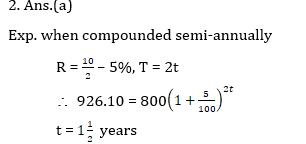3 . A man deposited Rs. 3,90,300 into the account of his two sons such that they will get equal money at their 18th birthday. Their ages today are 15 years and 13 years. Rate of interest is 4% per annum. Find the money deposited in their account.

(a) Rs. 130100, Rs. 260200

(b) Rs. 202000, Rs. 188300

(c) Rs. 152000, Rs. 238300

(d) Rs. 202800, Rs. 187500

(e) None of these4. The simple interest obtained on an amount of Rs. 45000 at the end of 4 years is Rs. 15300. What would be the approximate compound interest obtained on the same amount at the same rate of interest in the same period?(approximate)

(a) Rs. 18244

(b) Rs. 19500

(c) Rs. 16285

(d) Rs. 18566

(e) Rs. 17364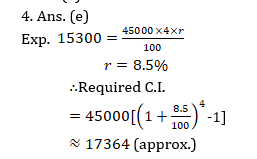5. A person invested equal amounts in two schemes A and B at the same rate of interest. Scheme A offers simple interest while scheme B offers compound interest. After two years he got Rs. 1920 from scheme A as interest and Rs. 2112 from scheme B as interest. If the rate of interest is increased by 4%, what will be the total interest after two years from both schemes?
(a) Rs. 4884.48
(b) Rs. 4888.48
(c) Rs. 4884.84
(d) Rs. 4384.48
(e) None of these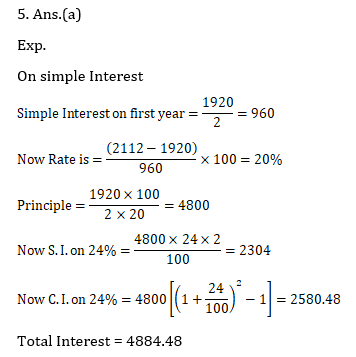6. Rs. 1750 is given at 14% SI per annum while Rs (1750 + P) is given @ 20% CI per annum. If the difference between both interests at the end of two years is Rs 830. Find P.

(a) 1100

(b) 1150

(c) 1250

(d) 1300

(e) 1350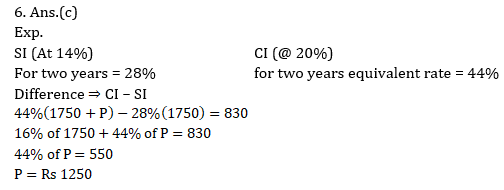7. A money lender lent out 25,000 in two parts, one at 8% and the other at 8.5% on S.I. If the total annual income on the amount is Rs. 2013.25, the money lent at 8% is

(a) Rs. 12500

(b) Rs. 6250

(c) Rs. 10000

(d) Rs. 18750

(e) None of these8. The simple interest on a certain sum for 8 months at 4% per annum is Rs. 129 less than the simple interest on the same sum for 15 months at 5% per annum. The sum is

(a) Rs. 2580

(b) Rs. 2400

(c) Rs. 2529

(d) Rs. 3600

(e) None of these9. The S.I on certain sum of money for 15 months at rate of 7.5% per annum exceed the S.I on same sum at 12.5% per annum for 8 months by Rs 3250 find sum?

(a) 160000

(b) 20000

(c) 170000

(d) 18000

(e) 312000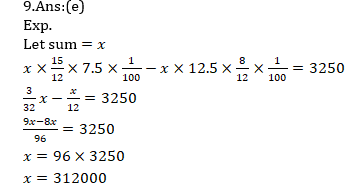10. A man invest money in three different scheme for 6 yr,10 yr& 12 yr at the rate of 10%,12% and 15% per annum at completion of each scheme he get same interest, what is ratio between their sum.

(a) 6:3:2

(b) 3:2:1

(c) 1:2:3

(d) 5:2:3

(e) 7:2:1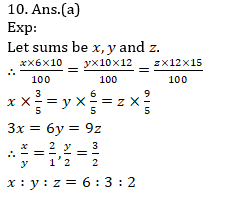### Online Mock Test Available on App as well as Web:### How to Access on App:-

1. Go to Playstore search Ambitious Baba or Click here to Install App